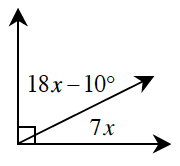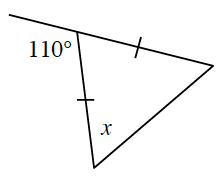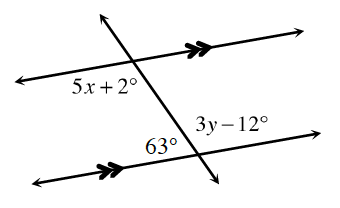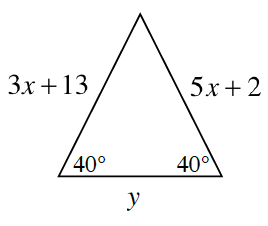### Home > CCG > Chapter 6 > Lesson 6.2.3 > Problem6-74

6-74.

For each diagram below, use geometric relationships to solve for the given variable(s). Check your answer.

1.The angle that is $18x-10º$ and the angle $7x$ are complementary.
Complementary means the angles add up to _____

1.Adjacent angle pairs that form a straight line are supplementary and base angles of an isosceles triangle are congruent.

$x=55º$

1.First solve for $y$, then solve for $x$.

$3y-12º+63º=180º$

1.Since the triangle is isosceles, start by solving for $x$.
Next, solve for the unknown interior angle.
Then use the Law of Sines to solve for $y$.

Refer to the Math Notes box in Lesson 5.3.2 if you need help remembering the Law of Sines.

$\textit{x} = \frac{11}{2}$

$\textit{y} = \frac{29.5\sin (100º)}{\sin (40º)}\approx 45.20$